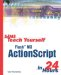# Numerical Operators and Functions

 I l @ ve RuBoard

In Hour 2, "Using the Script Editing Window," and Hour 3, "Learning to Program," you learned how variables can hold numbers. They can hold integers, such as 7, and floating point numbers , such as 1.3574.

#### Basic Operators

You also learned about basic numerical operators. Let's review them. The + and - symbols allow you to add and subtract numbers. The * symbol is for multiplication. The / symbol is for division.

You can also use += , -= , *= , and /= to simplify your code. For instance, the following two lines perform the same operation. In both cases, 7 is added to "a":

` a = a + 7; a += 7; `

#### Comparison Operators

You can compare two numbers with the == symbol to see whether they are equal. You can also use the < or > symbols as less than or greater than. The <= and >= symbols are for less than or equal to and greater than or equal to.

In ActionScript, you can freely compare integers and floating point numbers. For instance, a variable containing 7.2 would be greater than a variable containing 7.

#### Math.abs

The Math object is a collection of functions that work with numbers. For instance, the Math.abs function returns the absolute value of a number. This is simply the number without the positive or negative sign. So -7 returns 7, and 7 returns 7. Here is an example:

` trace(Math.abs(-7)); `

#### Math.round

If you are using floating point numbers, but want to display them to the user , you may decide to display them as integers instead of with the part of the number after the decimal point.

The most straightforward function for this is Math.round , which rounds a floating number up or down to the nearest integer. So 7.2 becomes 7, and 7.8 becomes 8.

` trace(Math.round(7.2)); trace(Math.round(7.8)); `

#### Math.ceil , Math.floor

Two other functions that convert floating point numbers to integers are Math.ceil and Math.floor . The first rounds a number up to the nearest integer, and the second rounds a number down. So the result of both these examples is 7:

` trace(Math.ceil(6.1)); trace(Math.floor(7.9)); `

#### Math.min , Math.max

You can compare two numbers and get the smallest or largest of the two using Math.min and Math.max . For instance, if "a" is 5, and "b" is 4, Math.min(a,b) returns 4, and Math.max(a,b) returns 5.

#### Math.pow

If you ever need to raise a number by a power, you can do so with the Math.pow function. The first parameter is the number, and the second is the power. So to get 4 to the third power, you can do this:

` trace(Math.pow(4,3)); `

#### Math.sqrt

You can also use numbers less than 1 as the power in Math.pow . This means that you can use Math.pow(x,.5) to get the square root of x. Or, you could use the shortcut function Math.sqrt .

` trace(Math.sqrt(4)); `
 I l @ ve RuBoardSams Teach Yourself Flash MX ActionScript in 24 Hours
ISBN: 0672323850
EAN: 2147483647
Year: 2002
Pages: 272

flylib.com © 2008-2017.
If you may any questions please contact us: flylib@qtcs.net